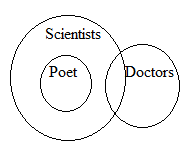# SSC CGL 2018 Practice Test Papers | Reasoning Ability (Day-47)

Dear Aspirants, Here we have given the Important SSC CGL Exam 2018 Practice Test Papers. Candidates those who are preparing for SSC CGL 2018 can practice these questions to get more confidence to Crack SSC CGL 2018 Examination.

[WpProQuiz 3400]

Click “Start Quiz” to attend these Questions and view Explanation

1) From the given alternatives, select the word which can be formed using the letters of the given word.

APPROXIMATION

a) PROXY

b) PAPER

c) MAID

d) AXIOM

2) A series is given, with one term missing. Choose the correct alternative from the given ones that will complete the series.

CTDSERFQGPH______

a) R

b) N

c) O

d) L

3) Some equations are solved on the basis of certain system. Find out the correct answer for the unsolved equation on that basis.

If 8 – 6 – 4 = 753, 3 – 6 – 9 = 258 then 2 – 7 – 5 = ?

a) 215

b) 164

c) 143

d) 102

4) Identify the diagram that best represents the relationship among Woman, Widow and Daughter.

a)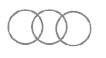b)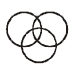c)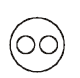d)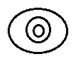5) A, B, C, D, E and F are sitting in a straight line facing North. B sits third to right of F and C sits second to right of B.A sits to the immediate left of D. What is D’s position with respect to F?

a) Second to the right

b) Third to the left

c) Immediate left

d) Immediate right

6) If  M – N means M is brother of N, M + N means M is sister of N, M & N means M is mother of N and M\$N means M is father of N, Which of the following means A is daughter of D?

a) C – D\$A&B

b) A+B-C&D

c) B&C+A&D

d) B – D&A-B

7) Which of the given answer figures will be the correct mirror image?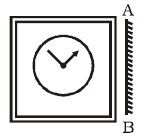a)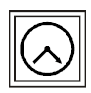b)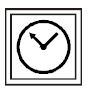c)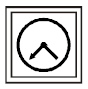d)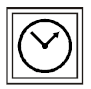8) Find the odd number/letter/ word pair from the given responses.

a) eeggiikk

b) ttuuwwxx

c) hhjjkkmm

d) nnpprrtt

9) If “CYCLE” is written as ELCCY then how “DWELL” is written in that language?

a) LLEDW

b) EDLLW

c) WLLDE

d) ELWDL

10) Statement:

All poets are Scientists.

Some scientists are Doctors.

Conclusion:

1. Some Doctors are not Poets.
2. Some Scientists are poets.

a) Only I follow

b) Only II follows

c) Both I and II follow

d) Neither I nor II follows.

AXIOM is the only word that can be formed from the word APPROXIMATION.

Alternate series:

i) C D E F G H

ii) T S R Q P O

8 – 6 – 4 = (8 – 1) | (6 – 1) | (4 – 1) = 753

3 – 6 – 9 = (3 – 1) | (6 – 1) | (9 – 1) = 258

2 – 7 – 5 = (2 – 1) | (7 – 1) | (5 – 1) = 164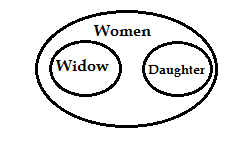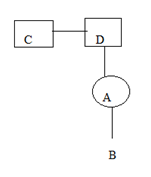Each letter is +2 from the previous letter and written twice.

Last three letters of the word are reversed and written as suffix to the word

CYCLE= CY   CLE

Last three letters are reversed => ELC

CODE: ELCCY

Similarly for DWELL,

DWELL= DW   LLE

CODE: LLEDW